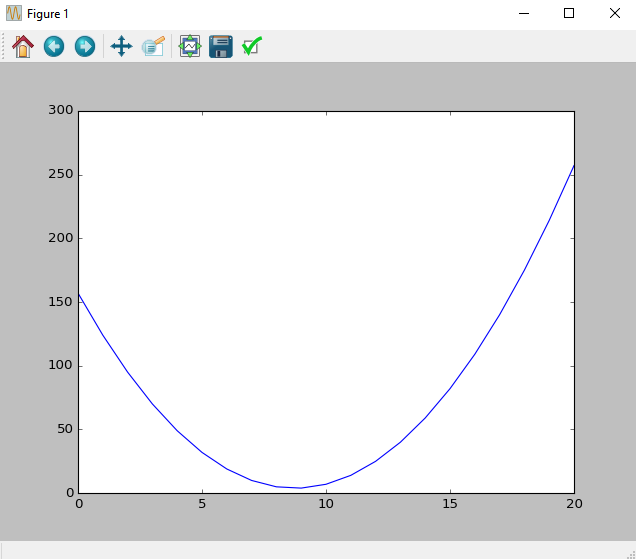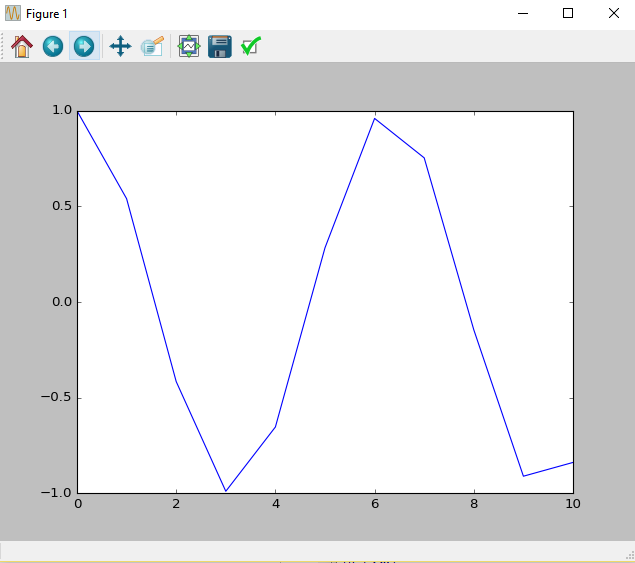By Matan Aspis | 8/4/2016 |

# Scipy Tutorial

Scipy:

First let's import the Scipy library with the following command:

``In : import scipy``

Define the polynomial (2*x ^2 +5*x+7):

``````In :p = scipy.poly1d([2, 5, 7])

In :p
Out: poly1d([2, 5, 7])``````
``````In :print p
2
2 x + 5 x + 7``````
``````In : x = np.linspace(-10, 10, 21)

In :plt.plot(p(x))
Out: [<matplotlib.lines.Line2D at 0x23750470>]``````Now we will import the library linalg of Scipy. This library will allow us to perform various mathematical operations on arrays. We'll start with actions on matrixes:
Note that a similar library can be imported from the library numpy, but the one that in scipy is faster and more recommended.

``In : from scipy import linalg``

Matrix multiplication:

Notice that if we'll multiply two-dimensional arrays (matrices) a simple multiplication. we'll get  a new vector in which each organ is the product of two elements in the same place respectively at matrices A, B. Lets preform some example:

``````In : A = np.full((3, 3), 3, dtype=int)

A
Out:
array([[3, 3, 3],
[3, 3, 3],
[3, 3, 3]])

In : B=np.arange(9).reshape(3,3)

B
Out:
array([[0, 1, 2],
[3, 4, 5],
[6, 7, 8]])

In : A*B
Out:
array([[ 0,  3,  6],
[ 9, 12, 15],
[18, 21, 24]])``````

In order to perform a valid matrix multiplication we use the following command:

``````In : A.dot(B)
Out:
array([[27, 36, 45],
[27, 36, 45],
[27, 36, 45]])``````

Matrix Transpose:

``````In : B.T
Out:
array([[0, 3, 6],
[1, 4, 7],
[2, 5, 8]])``````

Inverse:

``````In : I = np.array([[1, 2, 3],
[0, 5, 6],
[0, 0, 9]])

In : linalg.inv(I)

Out:
array([[ 1.        , -0.4       , -0.06666667],
[ 0.        ,  0.2       , -0.13333333],
[ 0.        ,  0.        ,  0.11111111]])``````

Determinant:

``````In : C = np.array([[1, 2, 3],
[7, 8, 6],
[4, 5, 6]])

In : linalg.det(C)
Out: -8.999999999999998``````

Norm:

``````In : C = np.array([[1, 2, 3],
[1, 4, 9],
[1, 16, 81]])``````

norm 1, frobenius, infinity :

``````In : linalg.norm(C, 1)----> Norm 1(The organs maximum sum at columns)
Out: 93.0

In : linalg.norm(C, 'fro')---->  Frobenius norm(Root sum of the squares of all  organs)
Out: 83.246621553069645

In : linalg.norm(C, np.inf)----> Infinity norm(The organs maximum sum at raws)
Out: 98.0
``````

interpolate :

We will import interp1d class which allows to perform interpolation on a one-dimensional arrays.

``In : from scipy.interpolate import interp1d``

``````In : x = np.linspace(0, 10,11)

In : y = np.cos(x)

In : plt.plot(x,y)
Out: [<matplotlib.lines.Line2D at 0x2c843cc0>``````For example, we will use two types of interpolations. First, a linear interpolation. And second, finer interpolation and more accurate one('cubic').

For this example, we created a new vector which divided  into more intermediate values.

``````In : x
Out: array([  0.,   1.,   2.,   3.,   4.,   5.,   6.,   7.,   8.,   9.,  10.])
In : x2 = np.linspace(0, 10,30)
y = np.cos(x)
I1 = interp1d(x, y)
I2 = interp1d(x, y, 'cubic')``````

I1,I2 behave like functions. We created I1,I2 accordind to vector x and we activate it on vector x2 which is finer. the function will returns us to the values of y by interpolation according to the vercotr x:

``````In : plt.plot(x2, np.cos(x2), 'o', x2, I1(x2), '-', x2, I2(x2), '--')
plt.legend(['data', 'linear', 'cubic'], loc='best')``````

Note that ("loc='best') Placing the legend in the most optimal place. for hiding less information from the graph as possible.scipy.stats:

This directory contains many statistical functions. They all operate under the same
Principles of the normal distribution:

First we need to import the following libraries:

``````In : import numpy as np

In : import matplotlib.pyplot as plt

In : from scipy import stats

In : %matplotlib
Using matplotlib backend: Qt4Agg``````

We will create a normal distribution (Expected value 0.25,standard deviation 1)  by use the following commands:

``````In : x = np.linspace(-5, 5, 150)

In : n = stats.norm(0.25, 1)

In : plt.plot(x, n.pdf(x),lw='3')``````Histogram:

We will use n.rvs function to creates 50 (An arbitrary number) random values at chosen distribution. In our case a normal distribution. The histogram divided into ten bins:

``````In : n = stats.norm()
x = n.rvs(50)
h = stats.histogram(x, numbins=10)
plt.bar(range(h.size), h, color='green')``````## Recent Stories

### Top DiscoverSDK Experts3355
Ashton Torrence
Web and Windows developer
GUI | Web and 11 more
View Profile3220
Mendy Bennett
Mobile | Ad Networks and 1 more
View Profile3060
Karen Fitzgerald
7 years in Cross-Platform development.
Mobile | Cross Platform Frameworks
View Profile
X

## Compare Products

Select up to three two products to compare by clicking on the compare icon () of each product.

{{compareToolModel.Error}}

### Now comparing:

{{product.ProductName | createSubstring:25}} X
Compare Now